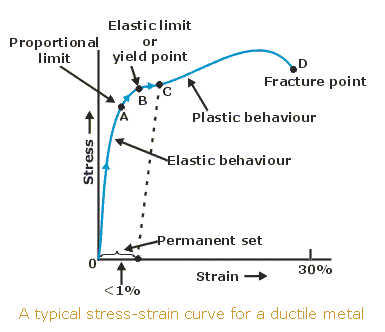# What is the definite definition of strength?

I have access to definite definition to terminology like stress(Pa), strain(dimensionless), ultimate strength(Pa), yield strength(Pa), etc. But I often get confused when someone just write "strength".

I give an example. Consider the following sentence:

"Titanium has high strength and high stiffness."

What is the strength referring here? Is it a measurable variable, or a qualitative description? If it is measurable, what is its unit, and in a typical stress-strain graph of a ductile material, where is this variable located?Mapes
Homework Helper
Gold Member
You're not alone in being confused. The word "strength" alone is ambiguous; it could refer to the yield strength in uniaxial tension, the ultimate strength in uniaxial tension, the shear strength, the fatigue strength, etc.

As an everyday (non-precise) term, it probably corresponds to the highest point on the engineering stress-strain diagram (not marked on yours), also known as the "ultimate strength," the point where a metal will begin to neck.

stewartcs
I have access to definite definition to terminology like stress(Pa), strain(dimensionless), ultimate strength(Pa), yield strength(Pa), etc. But I often get confused when someone just write "strength".

I give an example. Consider the following sentence:

"Titanium has high strength and high stiffness."

What is the strength referring here? Is it a measurable variable, or a qualitative description? If it is measurable, what is its unit, and in a typical stress-strain graph of a ductile material, where is this variable located?Used in that context the word strength generally means the material strength. So if you were to compare Titanium to Steel, you would see a higher overall strength (both yield and ultimate) for Titanium.

But like Mapes said...it's important to clearly define what one is referring to by using a qualifier like yield or ultimate...

CS

Mech_Engineer